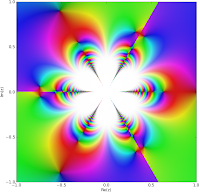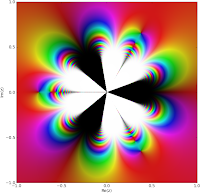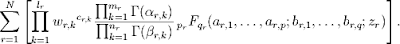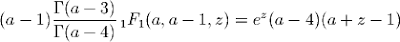## Monday, June 29, 2009

### Meijer G, more hypergeometric functions, fractional differentiation

My last update and the post before it detailed substantial improvements to the code for hypergeometric functions in mpmath, specifically the support for asymptotic expansions for 0F1, 1F1, 2F1, 2F0, plus the ability to evaluate hypergeometric-type formulas with singular parameters.

Over the past week-and-a-half I've done more work along the same lines. Importantly, I've implemented asymptotic expansions also for 1F2, 2F2 and 2F3 (commits 1, 2), so all hypergeometric functions of degree up to (2,3) now support fast evaluation for |z| → ∞ (1F0 also works, if anyone wonders -- it just happens to be a trivial case).

The next major remaining case is 3F2. It has a 1/z transformation, but this leaves |z| ≈ 1 which I don't know how to deal with. Does anyone who happens to be reading this know methods for evaluating 3F2 close to the unit circle? Taylor expansion around some point other than 0 works to some extent, but it's slow and in particular asymptotically slow close to z = 1, so not much help.

#### Bessel functions, etc.

As expected, the hypercomb function leads to very simple implementations of a large class of functions. I've now implemented the Whittaker, Struve, and Kelvin functions (they are called whitm, whitw, struveh, struvel, ber, bei, ker, kei). I've yet to update the orthogonal polynomials, but that shouldn't be much work. With this, I will have covered most of Bessel-type and hypergeometric-type functions listed on the Wolfram Functions site.

Speaking of Bessel functions, I also addressed most of the problems with their implementation in this commit. In particular, they can now be evaluated for huge arguments:

`>>> mp.dps = 30>>> print besselj(1, 10**20)-7.95068198242545016504555020084e-11>>> print chop(besselj(1, 10**20 * j))(0.0 + 5.17370851996688078482437428203e+43429448190325182754j)>>> print bessely(1,10**20)-6.69800904070342428527377044712e-12>>> print besselk(1,10**20)9.66424757155048856421325779143e-43429448190325182776`

This wasn't trivial, mainly because although hypercomb generally works, it sometimes becomes impossibly slow when computing functions straight from the definition. Basically: the function of interest might decrease exponentially, but internally it is computed by adding two nearly identical terms that grow exponentially, so the working precision and computation time increases exponentially. It's therefore still necessary to switch between different representations in different parts of the complex plane, and figuring that out involves some work. Some cases probably remain to be fixed.

As a followup to last week, I'll attach plots of J0(1/z3), Y0(1/z3) and K0(1/z3):The K plot unfortunately took very long time to finish -- almost an hour for 200,000 complex evaluations (J and Y were both much faster), so I'll probably have to optimize besselk a bit further.

#### Fractional derivatives

Another useful enhancement to the Bessel functions is that they can now be differentiated and integrated directly:

`>>> mp.dps = 30>>> print besselj(0, 3.5, derivative=2)0.419378462090785430440501275011>>> print diff(lambda x: besselj(0,x), 3.5, 2)0.419378462090785430440501275011>>> print besselj(0,3.5,derivative=-1) - besselj(0,2.5,derivative=-1)-0.244675206320579138611991019242>>> print quad(lambda x: besselj(0,x), [2.5, 3.5])-0.244675206320579138611991019242`

In fact, the representation for the derivatives works not just for integer orders (including negative values -- giving iterated integrals), but also for fractional or complex values. This led me to implement a general function differint for computing fractional order derivatives / integrals of arbitrary functions. (Commit.) It works essentially directly with the definition of the Riemann-Liouville differintegral.

It gives the same result as the special-purpose implementation for the Bessel function:

`>>> print besselj(0, 3.5, derivative=0.5)-0.436609427860836504473775239357>>> print differint(lambda x: besselj(0,x), 3.5, 0.5)-0.436609427860836504473775239357`

One neat application is iterated integration. The following gives a 5-fold integral of f(x) = exp(π x), along with the symbolic evaluation of the same as a check:

`>>> print differint(lambda x: exp(pi*x), 3.5, -5, x0=-inf)194.790546022218468869246881408>>> print exp(pi*3.5) / pi**5194.790546022218468869246881408`

Does anyone have any other interesting applications for fractional differentiation? I'd be interested in more examples to test with and possibly add to the documentation.

#### The Meijer G-function

At last, the Meijer G-function is implemented! (Commit.) Personally, I think this is something of a milestone for the mpmath project.

The Meijer G-function is very important because of its role in symbolic definite integration. Basically, definite integrals of Meijer G-functions (and even products of Meijer G-functions) just yield new Meijer G-functions; Mathematica and Maple therefore do many integrals by rewriting the input in terms of Meijer G-functions, applying Meijer G transformations, and converting the result back to simpler functions if possible.

Having the Meijer G-function in mpmath should be useful for anyone who wishes to implement a more powerful definite integrator in SymPy for example. It could also be useful for obtaining numerical values from integrals done by hand.

Looking around for examples to do stress testing with, I found a web page by Viktor Toth: Maple and Meijer's G-function: a numerical instability and a cure. His problem is to accurately evaluate G(-; 0; -1/2,-1,-3/2; -; x) for large real values of x. With my Meijer G-function implementation, I get:

`>>> mp.dps = 15>>> print meijerg([[],],[[-0.5,-1,-1.5],[]],10)4.89717497704114e-5>>> print meijerg([[],],[[-0.5,-1,-1.5],[]],100)1.09696661341118e-12>>> print meijerg([[],],[[-0.5,-1,-1.5],[]],1000)0.0>>> print meijerg([[],],[[-0.5,-1,-1.5],[]],10000)1.53249554086589e+54`

The third value should probably be small but not quite zero, and the last value is clearly bogus. Without looking at the details, the cause is almost certainly catastrophic cancellation of two huge terms. Fortunately, there is a cure for this:

`>>> print meijerg([[],],[[-0.5,-1,-1.5],[]],1000, check_cancellation=True)3.34093555343418e-33>>> print meijerg([[],],[[-0.5,-1,-1.5],[]],10000, check_cancellation=True)2.43925769071996e-94`

The cancellation check should probably be enabled by default, either to automatically redo the computation as above or at least to issue a warning. The only catch with this is that it might lead to unnecessary slowdown and/or annoyance for the user in some cases, so I'll have to investigate how common those cases are.

Incidentally, I also tried plugging the above two calculations into Mathematica 6.0. It takes a long time to finish (mpmath gives the result instantaneously) -- and returns values that are wrong!

`In:= u1 = MeijerG[{{},{0}},{{-1/2,-1,-3/2},{}},1000]                           3         1Out= MeijerG[{{}, {0}}, {{-(-), -1, -(-)}, {}}, 1000]                           2         2In:= u2 = MeijerG[{{},{0}},{{-1/2,-1,-3/2},{}},10000]                           3         1Out= MeijerG[{{}, {0}}, {{-(-), -1, -(-)}, {}}, 10000]                           2         2In:= Timing[N[u1,20]]Out= {8.90265, 0.0017597930166135139087}In:= Timing[N[u1,50]]                                                                    -33Out= {12.8231, 3.3409355534341801158987353523397047765918571151576 10   }In:=  Timing[N[u2,20]]                                      50Out= {59.017, -2.0782671663885270791 10  }In:= Timing[N[u2,50]]                                                                     22Out= {83.3753, -2.8700325450226332558088281915945389986057044454640 10  }In:= Timing[N[u2,120]]Out= {451.365, 2.439257690719956395903324691434088756714300374716395499173\                                                                    -94>      70196218529840153673260714339051464703903148052541923961351654 10   }`

That's several minutes for something mpmath did in less than a second, and with less intervention. So maybe Maple and Mathematica should copy my Meijer G-function code ;-)

#### Complex roots

A small and trivial, but quite convenient, new feature: the nthroot function can now compute any of the roots of a given number and not just the principal root. I also added root as an alias since I have lazy fingers. Like so:

`>>> for k in range(5):...     r = root(-12, 5, k)...     print chop(r**5), r...-12.0 (1.32982316461435 + 0.966173083818997j)-12.0 (-0.507947249855734 + 1.56330088863444j)-12.0 -1.64375182951723-12.0 (-0.507947249855734 - 1.56330088863444j)-12.0 (1.32982316461435 - 0.966173083818997j)`

While I was at it, I couldn't resist also implementing a function unitroots for computing all the nth roots of unity, optionally just the primitive roots, as well as a function cyclotomic for evaluating the nth cyclotomic polynomial. As it turns out, cyclotomic polynomials are not entirely trivial to evaluate both efficiently and in a numerically stable way -- see the source code for my solution. Commit here. A side effect is that I also implemented a powm1 function that accurately gives xy - 1 (possibly a useful complement to expm1 for other uses as well):

`>>> print power(2,1e-100)-10.0>>> print powm1(2, 1e-100)6.93147180559945e-101`

That will be all for now.

## Friday, June 19, 2009

### Massive hypergeometric update

[Update: as further proof that the asymptotic expansions are working, I've plotted 1/erfi(1/z3), Ai(1/z3) and Bi(1/z3) around 0:End of update.]

Today I committed a large patch to mpmath that significantly improves the state of hypergeometric functions. It's the result of about a week of work (plus some earlier research).

Perhaps most importantly, I've implemented the asymptotic expansions for 0F1 and 1F1 (the expansions for 2F1 were discussed in the previous post). They should now work for arbitrarily large arguments, as such:

`>>> from mpmath import *>>> mp.dps = 25>>> print hyp0f1(3,100)786255.7044208151250793134>>> print hyp0f1(3,100000000)4.375446848722142947128962e+8675>>> print hyp0f1(3,10**50)4.263410645749930620781402e+8685889638065036553022515>>> print hyp0f1(3,-10**50)-2.231890729584050840600415e-63>>> print hyp0f1(1000,1000+10**8*j)(-1.101783528465991973738237e+4700 - 1.520418042892352360143472e+4700j)>>> print hyp1f1(2,3,10**10)2.15550121570157969883678e+4342944809>>> print hyp1f1(2,3,-10**10)2.0e-20>>> print hyp1f1(2,3,10**10*j)(-9.750120502003974585202174e-11 - 1.746239245451213207369885e-10j)`

I also implemented 2F0 and U (Kummer's second function), mostly as a byproduct of the fact that 2F0 is needed for the asymptotic expansions of both 0F1 and 1F1. 2F0 is an interesting function: it is given by a divergent series (it converges only in special cases where it terminates after finitely many steps):However, it can be assigned a finite value for all z by expressing it in terms of U. Hence, mpmath can now compute the regularized sum of 2F0(a,b,z) for any arguments, say these ones:

`>>> print hyp2f0(5, -1.5, 4)(0.0000005877300438912428637649737 + 89.51091139854661783977495j)>>> print hyp2f0(5, -1.5, -4)102.594435262256516621777`

(This ought to be a novel feature; SciPy and GSL implement 2F0, but only special cases thereof, and Mathematica doesn't have a direct way to evaluate 2F0.)

It's the asymptotic case where z → 0, where a truncation of the 2F0 series can be used, that is used for the expansions at infinity of 0F1 and 1F1.

Back to 0F1 and 1F1, the asymptotic expansions of these functions are important because they permit many special functions to be evaluated efficiently for large arguments. So far I've fixed erf, erfc, erfi, airyai and airybi to take advantage of this fact (except for erf and erfc of a real variable, all these functions were previously slow even for a moderately large argument, say |z| > 100).

Examples that now work (well, some of them possibly theoretically worked before too, but probably required hours or ages of universe to finish):

`>>> print erf(10000+10000j)(1.000001659143196966967784 - 0.00003985971242709750831972313j)>>> print erfi(1000000000)2.526701758277367028229496e+434294481903251818>>> print erfc(1000-5j)(-1.27316023652348267063187e-434287 - 4.156805871732993710222905e-434288j)>>> print airyai(10**10)1.162235978298741779953693e-289529654602171>>> print airybi(10**10)1.369385787943539818688433e+289529654602165>>> print airyai(-10**10)0.0001736206448152818510510181>>> print airybi(-10**10)0.001775656141692932747610973>>> print airyai(10**10 * (1+j))(5.711508683721355528322567e-186339621747698 + 1.867245506962312577848166e-186339621747697j)>>> print airybi(10**10 * (1+j))(-6.559955931096196875845858e+186339621747689 - 6.822462726981357180929024e+186339621747690j)`

An essential addition in this patch is the function hypercomb which evaluates a linear combination of hypergeometric series, with gamma function and power weights:This is an extremely general function. Here is a partial list of functions that can be represented more or less directly by means of it:

• Regularized hypergeometric series

• The generalized incomplete gamma and beta functions (and their regularizations)

• Bessel functions

• Airy, Whittaker, Kelvin, Struve functions, etc

• Error functions

• Exponential, trigonometric and hyperbolic integrals

• Legendre, Chebyshev, Jacobi, Laguerre, Gegenbauer polynomials

• The Meijer G-function

That's most of Abramowitz & Stegun, and means that the remaining hypergeometric-type functions available in Mathematica or Maxima but absent in mpmath will be easy to implement in the near future. With these additions, mpmath will have the most comprehensive support for numerical hypergeometric-type functions of any open source software, and should be very close to Mathematica.

The most important virtue of hypercomb is not that it allows for more concise implementations of various hypergeometric-type functions, although that is a big advantage too. The main idea is that hypercomb can deal with singular subexpressions, and particularly with gamma function poles that cancel against singularities in the hypergeometric series. These cases are almost more common than the nonsingular cases in practice, and hypercomb saves the trouble of handling them in every separate function.

Thus, in principle, a numerically correct implementation of hypercomb leads to correct implementations of all the functions in the list above. It's not a silver bullet, of course. For example, if a particular but very common case of some common function triggers an expensive limit evaluation in hypercomb, then it's probably better to handle that case with special-purpose code. There are also most likely some bugs left in hypercomb, although by now I have tested a rather large set of examples; large enough to be confident that it works soundly.

To show an example of how it works, an implementation of the Bessel J function might look like this:

`def besj(n,z): z = mpmathify(z) h = lambda n: [([z/2],[n],[],[n+1],[],[n+1],-(z/2)**2)] return hypercomb(h, [n])>>> mp.dps = 30>>> print besselj(3,10.5)0.1632801643733625756462387>>> print besselj(-3,10.5)-0.1632801643733625756462387>>> print besj(3,10.5)0.1632801643733625756462387>>> print besj(-3,10.5)-0.1632801643733625756462387`

It gives the same value as the current Bessel J implementation in mpmath. Note that it works even when n is a negative integer, whereas naively evaluating the equation defining J(n,z) hits a gamma function pole and a division by zero in the hypergeometric series.

Thanks to the support for asymptotic expansions, this implementation (unlike the current besselj will also work happily with large arguments):

`>>> print besj(3,1e9)0.00000521042254280399021290721662036`

I'm soon going to fix all the Bessel functions in mpmath along these lines, as I already did with erf, airyai, etc.

Here is another, more involved example (quoting directly from the docstring for hypercomb). The following evaluateswith a=1, z=3. There is a zero factor, two gamma function poles, and the 1F1 function is singular; all singularities cancel out to give a finite value:

`>>> from mpmath import *>>> mp.dps = 15>>> print hypercomb(lambda a: [([a-1],,[a-3],[a-4],[a],[a-1],3)], )-180.769832308689>>> print -9*exp(3)-180.769832308689`

With some tweaks, the same code perhaps with a few tweaks could be used to symbolically evaluate hypergeometric-type functions (in the sense of rewriting them as pure hypergeometric series, and then possibly evaluating those symbolically as a second step). Symbolic support for hypergeometric functions is a very interesting (and hard) problem, and extremely important for computer algebra, but unfortunately I don't have time to work on that at the moment (there is more than enough to do just on the numerical side).

## Thursday, June 11, 2009

### Hypergeometric 2F1, incomplete beta, exponential integrals

One of the classes of functions I'm currently looking to improve in mpmath is the hypergeometric functions; particularly 1F1 (equivalently the incomplete gamma function) and the Gauss hypergeometric function 2F1.

For example, the classical orthogonal polynomials (Legendre, Chebyshev, Jacobi) are instances of 2F1 with certain integer parameters, and 2F1 with noninteger parameters allows for generalization of these functions to noninteger orders. Other functions that can be reduced to 2F1 include elliptic integrals (though mpmath uses AGM for these). With a good implementation of 2F1, these functions can be implemented very straightforwardly without a lot of special-purpose code to handle all their corner cases.

Numerical evaluation of 2F1 is far from straightforward, and the hyp2f1 function in mpmath used to be quite fragile. The hypergeometric series only converges for |z| < 1, and rapidly only for |z| << 1. There is a transformation that replaces z with 1/z, but this leaves arguments close to the unit circle which must be handled using further transformations. As if things weren't complicated enough, the transformations involve gamma function factors that often become singular even when the value of 2F1 is actually finite, and obtaining the correct finite value involves appropriately cancelling the singularities against each other.

After about two days of work, I've patched the 2F1 function in mpmath to the point where it should finally work for all complex values of a, b, c, z (see commits here). I'm not going to bet money that there isn't some problematic case left unhandled, but I've done tests for many of the special cases now.

The following is a very simple example that previously triggered a division by zero but now works:
`>>> print hyp2f1(3,-1,-1,0.5)2.5`

The following previously returned something like -inf + nan*j, due to incorrect handling of gamma function poles, but now works:
`>>> print hyp2f1(1,1,4,3+4j)(0.492343840009635 + 0.60513406166124j)>>> print (717./1250-378j/625)-(6324./15625-4032j/15625)*log(-2-4j)  # Exact(0.492343840009635 + 0.60513406166124j)`

Evaluation close to the unit circle used to be completely broken, but should be fine now. A simple test is to integrate along the unit circle:

`>>> mp.dps = 25>>> a, b, c = 1.5, 2, -4.25>>> print quad(lambda z: hyp2f1(a,b,c,exp(j*z)), [pi/2, 3*pi/2])(14.97223917917104676241015 + 1.70735170126956043188265e-24j)`

Mathematica gives the same value:
`In:= NIntegrate[Hypergeometric2F1[3/2,2,-17/4,Exp[I z]],    {z, Pi/2, 3Pi/2}, WorkingPrecision->25]                                                                   -26Out= 14.97223917917104676241014 - 3.514976640925973851950882 10    I`

Finally, evaluation at the singular point z = 1 now works and knows whether the result is finite or infinite:
`>>> print hyp2f1(1, 0.5, 3, 1)1.333333333333333333333333>>> print hyp2f1(1, 4.5, 3, 1)+inf`

As a consequence of these improvements, several mpmath functions (such as the orthogonal polynomials) should now work for almost all complex parameters as well.

The improvements to 2F1 also pave the way for some new functions. One of the many functions that can be reduced to 2F1 is the generalized incomplete beta function:An implementation of this function (betainc(a,b,x1,x2)) is now available in mpmath trunk. I wrote the basics of this implementation a while back, but it was nearly useless without the recent upgrades to 2F1. Evaluating the incomplete beta function with various choices of parameters proved useful to identify and fix some corner cases in 2F1.

One important application of the incomplete beta integral is that, when regularized, it is the cumulative distribution function of the beta distribution. As a sanity check, the following code successfully reproduces the plot of several beta CDF:s on the Wikipedia page for the beta distribution (I even got the same colors!):

`def B(a,b):    return lambda t: betainc(a,b,0,t,regularized=True)plot([B(1,3),B(0.5,0.5),B(5,1),B(2,2),B(2,5)], [0,1])`The betainc function is superior to manual numerical integration because of the numerically hairy singularities that occur at x = 0 and x = 1 for some choices of parameters. Thanks to having a good 2F1 implementation, betainc gives accurate results even in those cases.

The betainc function also provides an appropriate analytic continuation of the beta integral, internally via the analytic continuation of 2F1. Thus the beta integral can be evaluated outside of the standard interval [0,1]; for parameters where the integrand is singular at 0 or 1, this is in the sense of a contour that avoids the singularity.

It is interesting to observe how the integration introduces branch cuts; for example, in the following plot, you can see that 0 is a branch point when the first parameter is fractional and 1 is a branch point when the second parameter is fractional (when both are positive integers, the beta integral is just a polynomial, so it then behaves nicely):

`# blue, red, greenplot([B(2.5,2), B(3,1.5), B(3,2)], [-0.5,1.5], [-0.5,1.5])`To check which integration path betainc "uses", we can compare with numerical integration. For example, to integrate from 0 to 1.5, we can choose a contour that passes through +i (in the upper half plane) or -i (in the lower half plane):

`>>> mp.dps = 25>>> print betainc(3, 1.5, 0, 1.5)(0.152380952380952380952381 + 0.4023774302466306150757186j)>>> print quad(lambda x: x**2*(1-x)**0.5, [0, j, 1.5])(0.152380952380952380952381 - 0.4023774302466306150757186j)>>> print quad(lambda x: x**2*(1-x)**0.5, [0, -j, 1.5])(0.152380952380952380952381 + 0.4023774302466306150757186j)`

The sign of the imaginary part shows that betainc gives the equivalent of a contour through the lower half plane. The convention turns out to agree with that used by Mathematica:

`In:= Beta[0, 1.5, 3, 1.5]Out= 0.152381 + 0.402377 I`

I'll round things up by noting that I've also implemented the generalized exponential integral (the En-function) in mpmath as expint(n,z). A sample:

`>>> print expint(2, 3.5)0.005801893920899125522331056>>> print quad(lambda t: exp(-3.5*t)/t**2, [1,inf])0.005801893920899125522331056`

The En-function is based on the incomplete gamma function, which is based on the hypergeometric series 1F1. These functions are still slow and/or inaccurate for certain arguments (in particular, for large ones), so they will require improvements along the lines of those for 2F1. Stay tuned for progress.

In other news, mpmath 0.12 should be in both SymPy and Sage soon. With this announcement I'm just looking for an excuse to tag this post with both 'sympy' and 'sage' so it will show up on both Planet SymPy and Planet Sage :-) Posts purely about mpmath development should be relevant to both audiences though, I hope.

## Tuesday, June 9, 2009

### Mpmath 0.12 released

I'll quote the mailing list announcement:

Mpmath version 0.12 is now available from the website:

http://pypi.python.org/pypi/mpmath/0.12

Mpmath is a pure-Python library for arbitrary-precision floating-point arithmetic that implements an extensive set of mathematical functions. It can be used as a standalone library or via SymPy (http://code.google.com/p/sympy/).

Version 0.12 mostly contains bug fixes and speed improvements. New features include various special functions from analytic number theory, Newton's method as an option for root-finding, and more versatile printing of intervals. It is now also possible to create multiple working contexts each with its own precision. Finally, mpmath now recognizes being installed in Sage and will automatically wrap Sage's fast integer arithmetic if available.

For more details, see the changelog:

Bug reports and other comments are welcome at the issue tracker at
http://code.google.com/p/mpmath/issues/list or the mpmath mailing list:

I've previously blogged about some of the new features:

Computing generalized Bell numbers
Approximate prime counting
Fun with zeta functions

See those posts or the documentation for many examples.

## Saturday, June 6, 2009

### Cython mpmath performance, part 2

A followup to the previous post. Turns out I had left a temporary integer variable undeclared, which slowed things down unnecessarily. After adding a simple cdef, the code runs a bit faster: at 53 bits, an addition now takes 850 ns, a multiplication 920 ns; a complex addition takes 1.25 µs and a complex multiplication takes 2.15 µs.

I've also implemented division and square root. To continue the benchmarking started in the previous post:

`Real division, x/y       mpmath    cython    sage53     23.7 µs   1.49 µs   854 ns100    24.6 µs   1.84 µs   1.17 µs333    27 µs     2.69 µs   2.1 µs3333   70.3 µs   44 µs     42.8 µsReal square root, x.sqrt()53     21.1 µs   1.75 µs   1.48 µs100    22.5 µs   2.23 µs   1.68 µs333    32 µs     3.81 µs   3.11 µs3333   65.2 µs   35.3 µs   33.1 µs`

Division is a bit slow compared Sage, but it might be possible to improve. As with the other arithmetic operations, the speedup vs ordinary mpmath is still more than a factor 10 at low precision.

Further, here is a benchmark of the new gamma function algorithm (mentioned in my talk at SD15), which is an order of magnitude faster than the algorithms currently used by both mpmath and MPFR. I had already written a pure Python implementation, which was quite fast, but it's insanely fast in Cython -- at really low precision it's even faster than the exponential function (although this fact probably indicates that the exponential function could be streamlined further). It's still half as fast as exp at 333-bit precision:

`Real gamma function, x.gamma()   (gamma(x) in mpmath)       mpmath    cython    sage53     332 µs    8.6 µs    195 µs100    434 µs    12.9 µs   257 µs333    1.63 ms   52 µs     975 µs3333   90.9 ms   9.95 ms   347 ms`

Yes, that is a whopping 20,000 hundred-digit gamma functions per second!

Making the Cython code fully usable as an mpmath backend might require another week or two of work. This estimate includes rewriting utility code in Cython, but not porting transcendental functions. (The main reason why this will take longer is that I don't just want to translate the existing code, but redo the implementations from scratch to make everything uniformly faster.)

I'm not working full time on Cython mpmath backend; in parallel, I'm working on the mpmath wrapper code for Sage (some of which is ready and has been posted to the Sage trac) and on high-level implementations of various special functions. The new functions I have written partial implementations for so far include the generalized exponential integral (En), incomplete beta function, Hurwitz zeta function with derivatives, Appell hypergeometric functions of two variables, and matrix sqrt/exp/log/cos/sin. If anyone feels that their favorite special function is missing from Sage and/or mpmath, I'm taking requests :-)

I've also done little bit of work fixing hypergeometric functions for the hard cases, but really haven't made much progress on this so far (this is a substantial project which will take some time).

By the way, there should be a new mpmath release any day now. I mostly need to review the documentation and do some minor cleanup.